# Capacitors.ppt

31 de May de 2023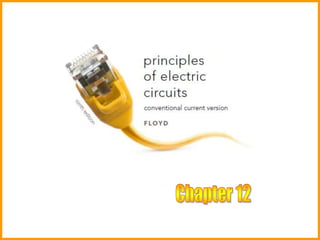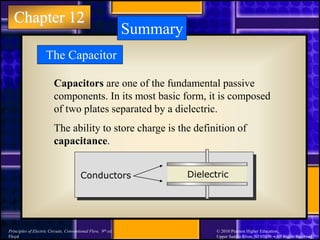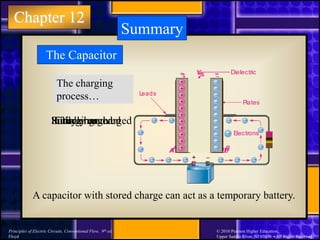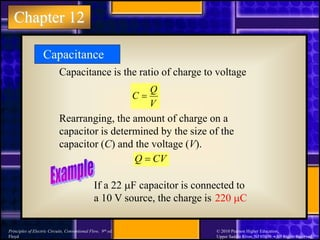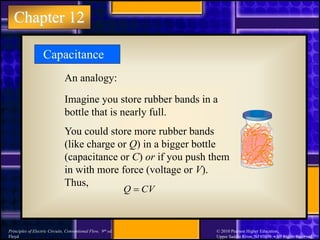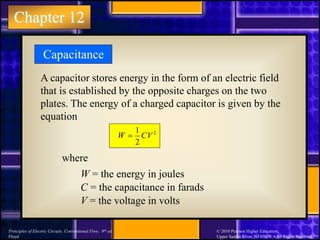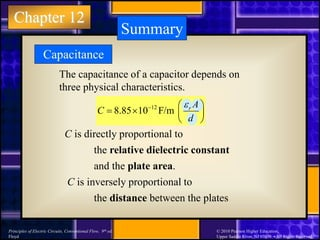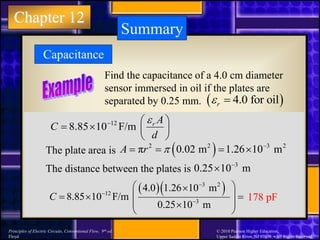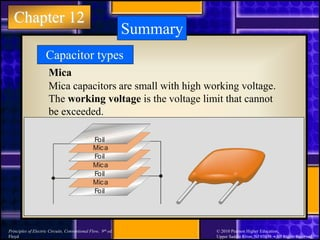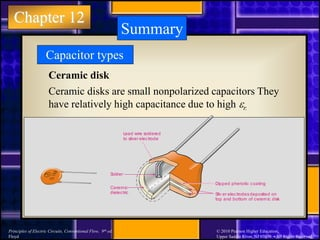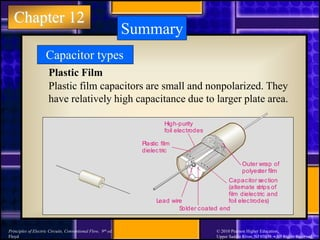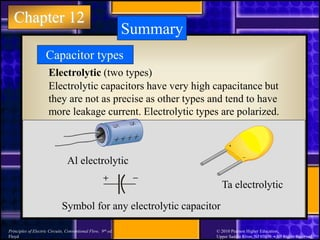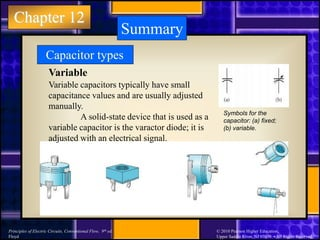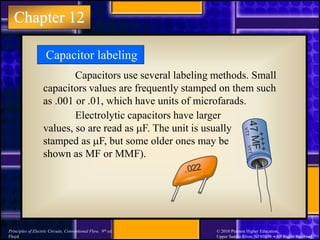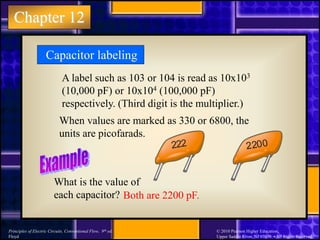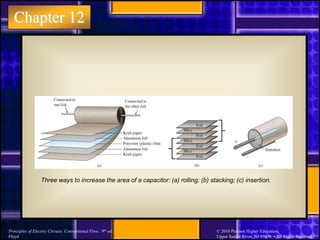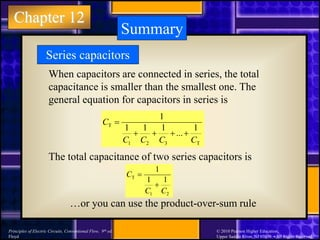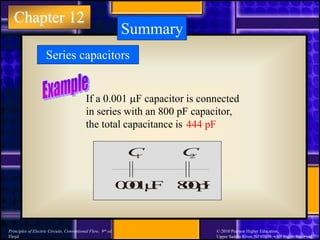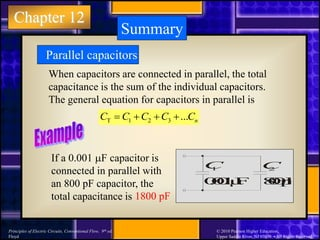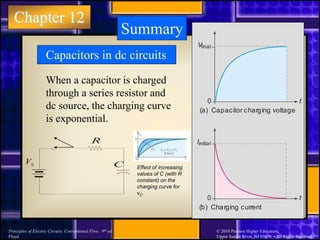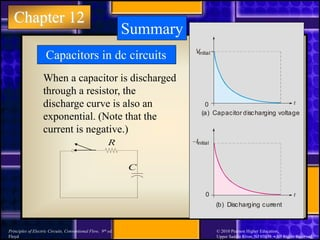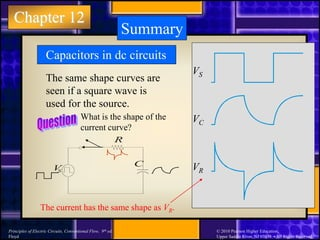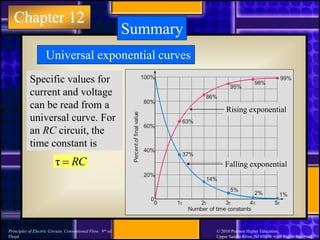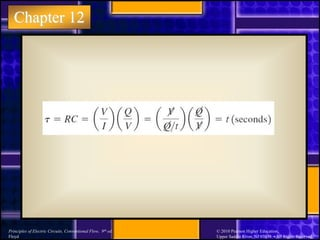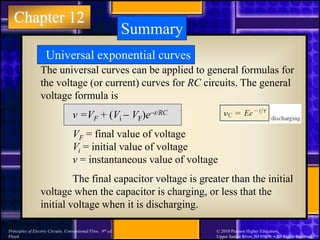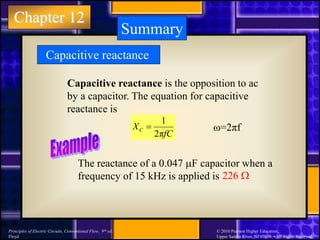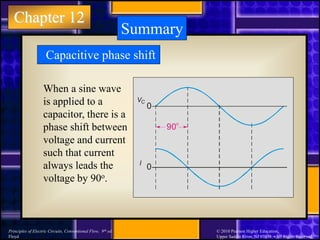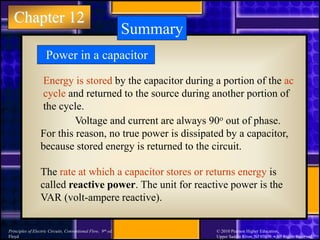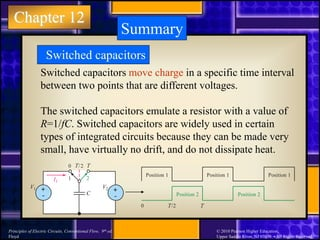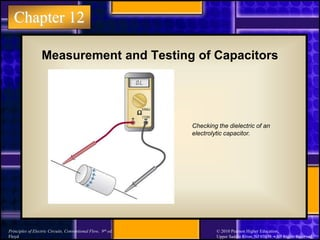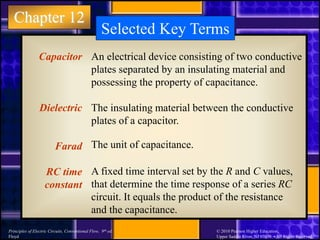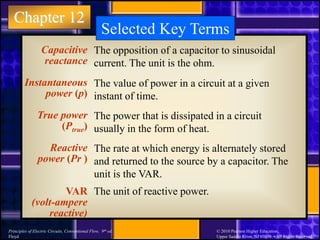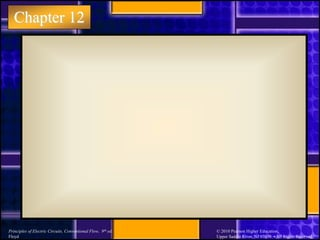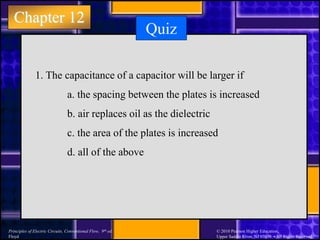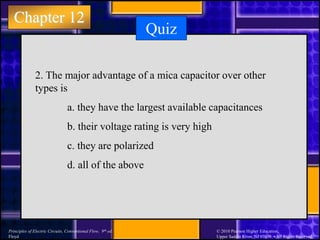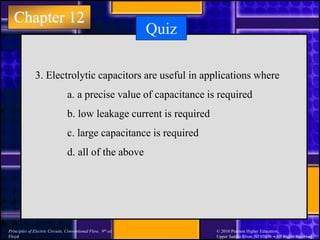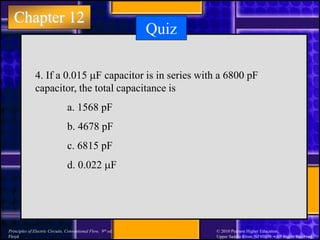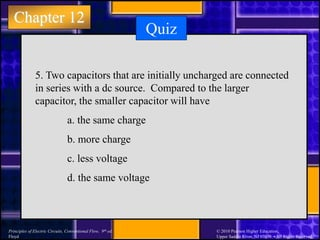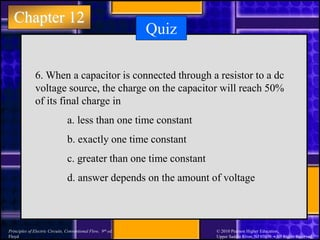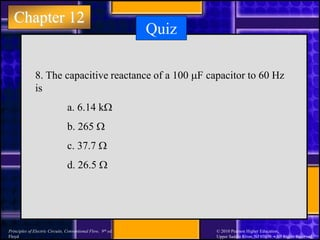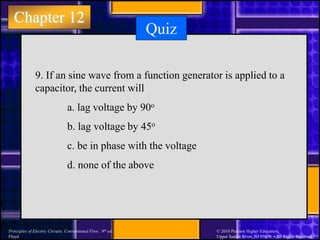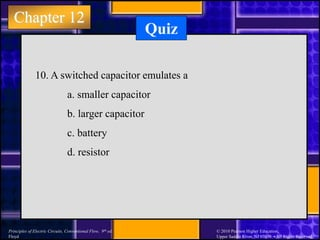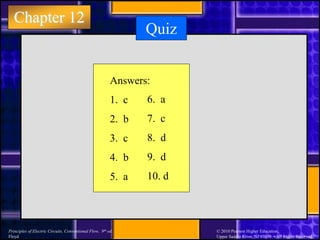1 de 44

### Capacitors.ppt

• 2. Chapter 12 Principles of Electric Circuits, Conventional Flow, 9th ed. Floyd © 2010 Pearson Higher Education, Upper Saddle River, NJ 07458. • All Rights Reserved Summary The Capacitor Capacitors are one of the fundamental passive components. In its most basic form, it is composed of two plates separated by a dielectric. The ability to store charge is the definition of capacitance. Dielectric Conductors
• 3. Chapter 12 Principles of Electric Circuits, Conventional Flow, 9th ed. Floyd © 2010 Pearson Higher Education, Upper Saddle River, NJ 07458. • All Rights Reserved Summary The Capacitor Dielectric Plates Leads Electrons B A     + + + +   + + + +  Initially uncharged +  B A        + + +               + + + + Charging +  B A VS + + + + + + + + + + +            Fully charged B A VS  +  +  +  +  +  +  +  +  +  +  + Source removed The charging process… A capacitor with stored charge can act as a temporary battery.
• 4. Chapter 12 Principles of Electric Circuits, Conventional Flow, 9th ed. Floyd © 2010 Pearson Higher Education, Upper Saddle River, NJ 07458. • All Rights Reserved Capacitance is the ratio of charge to voltage Q C V  Rearranging, the amount of charge on a capacitor is determined by the size of the capacitor (C) and the voltage (V). Q CV  If a 22 mF capacitor is connected to a 10 V source, the charge is 220 mC Capacitance
• 5. Chapter 12 Principles of Electric Circuits, Conventional Flow, 9th ed. Floyd © 2010 Pearson Higher Education, Upper Saddle River, NJ 07458. • All Rights Reserved Capacitance An analogy: Imagine you store rubber bands in a bottle that is nearly full. You could store more rubber bands (like charge or Q) in a bigger bottle (capacitance or C) or if you push them in with more force (voltage or V). Thus, Q CV 
• 6. Chapter 12 Principles of Electric Circuits, Conventional Flow, 9th ed. Floyd © 2010 Pearson Higher Education, Upper Saddle River, NJ 07458. • All Rights Reserved A capacitor stores energy in the form of an electric field that is established by the opposite charges on the two plates. The energy of a charged capacitor is given by the equation Capacitance 2 2 1 CV W  where W = the energy in joules C = the capacitance in farads V = the voltage in volts
• 7. Chapter 12 Principles of Electric Circuits, Conventional Flow, 9th ed. Floyd © 2010 Pearson Higher Education, Upper Saddle River, NJ 07458. • All Rights Reserved The capacitance of a capacitor depends on three physical characteristics. Summary 12 8.85 10 F/m r A C d           C is directly proportional to and the plate area. the relative dielectric constant C is inversely proportional to the distance between the plates Capacitance
• 8. Chapter 12 Principles of Electric Circuits, Conventional Flow, 9th ed. Floyd © 2010 Pearson Higher Education, Upper Saddle River, NJ 07458. • All Rights Reserved 12 8.85 10 F/m r A C d           Summary Find the capacitance of a 4.0 cm diameter sensor immersed in oil if the plates are separated by 0.25 mm. The plate area is The distance between the plates is   4.0 for oil r      3 2 12 3 4.0 1.26 10 m 8.85 10 F/m 0.25 10 m C                 3 0.25 10 m   178 pF   2 2 3 2 π 0.02 m 1.26 10 m A r       Capacitance
• 9. Chapter 12 Principles of Electric Circuits, Conventional Flow, 9th ed. Floyd © 2010 Pearson Higher Education, Upper Saddle River, NJ 07458. • All Rights Reserved Summary Capacitor types Mica Mica Foil Foil Mica Foil Foil Mica Foil Mica capacitors are small with high working voltage. The working voltage is the voltage limit that cannot be exceeded.
• 10. Chapter 12 Principles of Electric Circuits, Conventional Flow, 9th ed. Floyd © 2010 Pearson Higher Education, Upper Saddle River, NJ 07458. • All Rights Reserved Summary Capacitor types Ceramic disk Solder Lead wire soldered to silver electrode Ceramic dielectric Dipped phenolic coating Silv er electrodes deposited on top and bottom of ceramic disk Ceramic disks are small nonpolarized capacitors They have relatively high capacitance due to high r.
• 11. Chapter 12 Principles of Electric Circuits, Conventional Flow, 9th ed. Floyd © 2010 Pearson Higher Education, Upper Saddle River, NJ 07458. • All Rights Reserved Summary Capacitor types Plastic Film Lead wire High-purity foil electrodes Plastic film dielectric Outer wrap of polyester film Capacitor section (alternate strips of film dielectric and foil electrodes) S older coated end Plastic film capacitors are small and nonpolarized. They have relatively high capacitance due to larger plate area.
• 12. Chapter 12 Principles of Electric Circuits, Conventional Flow, 9th ed. Floyd © 2010 Pearson Higher Education, Upper Saddle River, NJ 07458. • All Rights Reserved Summary Capacitor types Electrolytic (two types) Symbol for any electrolytic capacitor Al electrolytic + _ Ta electrolytic Electrolytic capacitors have very high capacitance but they are not as precise as other types and tend to have more leakage current. Electrolytic types are polarized.
• 13. Chapter 12 Principles of Electric Circuits, Conventional Flow, 9th ed. Floyd © 2010 Pearson Higher Education, Upper Saddle River, NJ 07458. • All Rights Reserved Summary Capacitor types Variable Variable capacitors typically have small capacitance values and are usually adjusted manually. A solid-state device that is used as a variable capacitor is the varactor diode; it is adjusted with an electrical signal. Symbols for the capacitor: (a) fixed; (b) variable.
• 14. Chapter 12 Principles of Electric Circuits, Conventional Flow, 9th ed. Floyd © 2010 Pearson Higher Education, Upper Saddle River, NJ 07458. • All Rights Reserved Capacitor labeling Capacitors use several labeling methods. Small capacitors values are frequently stamped on them such as .001 or .01, which have units of microfarads. + + + + V TT VT T 4 7 M F .022 Electrolytic capacitors have larger values, so are read as mF. The unit is usually stamped as mF, but some older ones may be shown as MF or MMF).
• 15. Chapter 12 Principles of Electric Circuits, Conventional Flow, 9th ed. Floyd © 2010 Pearson Higher Education, Upper Saddle River, NJ 07458. • All Rights Reserved A label such as 103 or 104 is read as 10x103 (10,000 pF) or 10x104 (100,000 pF) respectively. (Third digit is the multiplier.) Capacitor labeling When values are marked as 330 or 6800, the units are picofarads. What is the value of each capacitor? Both are 2200 pF. 222 2200
• 16. Chapter 12 Principles of Electric Circuits, Conventional Flow, 9th ed. Floyd © 2010 Pearson Higher Education, Upper Saddle River, NJ 07458. • All Rights Reserved Three ways to increase the area of a capacitor: (a) rolling; (b) stacking; (c) insertion.
• 17. Chapter 12 Principles of Electric Circuits, Conventional Flow, 9th ed. Floyd © 2010 Pearson Higher Education, Upper Saddle River, NJ 07458. • All Rights Reserved Summary Series capacitors When capacitors are connected in series, the total capacitance is smaller than the smallest one. The general equation for capacitors in series is T 1 2 3 T 1 1 1 1 1 ... C C C C C      The total capacitance of two series capacitors is T 1 2 1 1 1 C C C   …or you can use the product-over-sum rule
• 18. Chapter 12 Principles of Electric Circuits, Conventional Flow, 9th ed. Floyd © 2010 Pearson Higher Education, Upper Saddle River, NJ 07458. • All Rights Reserved Summary Series capacitors If a 0.001 mF capacitor is connected in series with an 800 pF capacitor, the total capacitance is 444 pF 0 . 0 0 1 µ F 8 0 0 p F C 1 C 2
• 19. Chapter 12 Principles of Electric Circuits, Conventional Flow, 9th ed. Floyd © 2010 Pearson Higher Education, Upper Saddle River, NJ 07458. • All Rights Reserved Summary Parallel capacitors When capacitors are connected in parallel, the total capacitance is the sum of the individual capacitors. The general equation for capacitors in parallel is T 1 2 3 ... n C C C C C     1800 pF If a 0.001 mF capacitor is connected in parallel with an 800 pF capacitor, the total capacitance is 0 . 0 0 1 µ F 8 0 0 p F C 1 C 2
• 20. Chapter 12 Principles of Electric Circuits, Conventional Flow, 9th ed. Floyd © 2010 Pearson Higher Education, Upper Saddle River, NJ 07458. • All Rights Reserved Summary Capacitors in dc circuits When a capacitor is charged through a series resistor and dc source, the charging curve is exponential. C R Iinitial t 0 (b) Charging current Vfinal t 0 (a) Capacitor charging voltage Effect of increasing values of C (with R constant) on the charging curve for vC.
• 21. Chapter 12 Principles of Electric Circuits, Conventional Flow, 9th ed. Floyd © 2010 Pearson Higher Education, Upper Saddle River, NJ 07458. • All Rights Reserved Summary Capacitors in dc circuits When a capacitor is discharged through a resistor, the discharge curve is also an exponential. (Note that the current is negative.) t t Iinitial 0 (b) Discharging current Vinitial 0 (a) Capacitor discharging voltage C R
• 22. Chapter 12 Principles of Electric Circuits, Conventional Flow, 9th ed. Floyd © 2010 Pearson Higher Education, Upper Saddle River, NJ 07458. • All Rights Reserved Summary Capacitors in dc circuits The same shape curves are seen if a square wave is used for the source. VS VC VR C R V S What is the shape of the current curve? The current has the same shape as VR.
• 23. Chapter 12 Principles of Electric Circuits, Conventional Flow, 9th ed. Floyd © 2010 Pearson Higher Education, Upper Saddle River, NJ 07458. • All Rights Reserved Summary Universal exponential curves Specific values for current and voltage can be read from a universal curve. For an RC circuit, the time constant is τ RC  100% 80% 60% 40% 20% 0 0 1t 2t 3t 4t 5t 99% 98% 95% 86% 63% 37% 14% 5% 2% 1% Number of time constants Percent of final value Rising exponential Falling exponential
• 25. Chapter 12 Principles of Electric Circuits, Conventional Flow, 9th ed. Floyd © 2010 Pearson Higher Education, Upper Saddle River, NJ 07458. • All Rights Reserved Summary The universal curves can be applied to general formulas for the voltage (or current) curves for RC circuits. The general voltage formula is v =VF + (Vi  VF)et/RC VF = final value of voltage Vi = initial value of voltage v = instantaneous value of voltage The final capacitor voltage is greater than the initial voltage when the capacitor is charging, or less that the initial voltage when it is discharging. Universal exponential curves
• 26. Chapter 12 Principles of Electric Circuits, Conventional Flow, 9th ed. Floyd © 2010 Pearson Higher Education, Upper Saddle River, NJ 07458. • All Rights Reserved Summary Capacitive reactance Capacitive reactance is the opposition to ac by a capacitor. The equation for capacitive reactance is 1 2π C X fC  The reactance of a 0.047 mF capacitor when a frequency of 15 kHz is applied is 226 W ω=2πf
• 27. Chapter 12 Principles of Electric Circuits, Conventional Flow, 9th ed. Floyd © 2010 Pearson Higher Education, Upper Saddle River, NJ 07458. • All Rights Reserved Summary Capacitive phase shift When a sine wave is applied to a capacitor, there is a phase shift between voltage and current such that current always leads the voltage by 90o. VC 0 I 0 90 o
• 28. Chapter 12 Principles of Electric Circuits, Conventional Flow, 9th ed. Floyd © 2010 Pearson Higher Education, Upper Saddle River, NJ 07458. • All Rights Reserved Summary Power in a capacitor Voltage and current are always 90o out of phase. For this reason, no true power is dissipated by a capacitor, because stored energy is returned to the circuit. The rate at which a capacitor stores or returns energy is called reactive power. The unit for reactive power is the VAR (volt-ampere reactive). Energy is stored by the capacitor during a portion of the ac cycle and returned to the source during another portion of the cycle.
• 29. Chapter 12 Principles of Electric Circuits, Conventional Flow, 9th ed. Floyd © 2010 Pearson Higher Education, Upper Saddle River, NJ 07458. • All Rights Reserved Summary Switched capacitors Switched capacitors move charge in a specific time interval between two points that are different voltages. The switched capacitors emulate a resistor with a value of R=1/fC. Switched capacitors are widely used in certain types of integrated circuits because they can be made very small, have virtually no drift, and do not dissipate heat. V1 V2 I1 C 1 0 T/2 2 T Position 1 Position 1 Position 1 Position 2 Position 2 0 T T/2 + + - -
• 30. Chapter 12 Principles of Electric Circuits, Conventional Flow, 9th ed. Floyd © 2010 Pearson Higher Education, Upper Saddle River, NJ 07458. • All Rights Reserved Measurement and Testing of Capacitors Checking the dielectric of an electrolytic capacitor.
• 31. Chapter 12 Principles of Electric Circuits, Conventional Flow, 9th ed. Floyd © 2010 Pearson Higher Education, Upper Saddle River, NJ 07458. • All Rights Reserved Selected Key Terms Capacitor Dielectric Farad RC time constant An electrical device consisting of two conductive plates separated by an insulating material and possessing the property of capacitance. The insulating material between the conductive plates of a capacitor. The unit of capacitance. A fixed time interval set by the R and C values, that determine the time response of a series RC circuit. It equals the product of the resistance and the capacitance.
• 32. Chapter 12 Principles of Electric Circuits, Conventional Flow, 9th ed. Floyd © 2010 Pearson Higher Education, Upper Saddle River, NJ 07458. • All Rights Reserved Capacitive reactance Instantaneous power (p) True power (Ptrue) Reactive power (Pr ) VAR (volt-ampere reactive) The value of power in a circuit at a given instant of time. The power that is dissipated in a circuit usually in the form of heat. The opposition of a capacitor to sinusoidal current. The unit is the ohm. Selected Key Terms The rate at which energy is alternately stored and returned to the source by a capacitor. The unit is the VAR. The unit of reactive power.